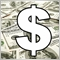# Need some help with this37

hello guys i am trying to understand the time function for a while now but still having some complications

actually i am working on an indicator that show a line 10 pips above and below the candle open price

it will show the line when the price open is below or above the exponential moving average.

what i want is the line to be visible only when the sever time is at 3 am , means the indicator only show these line when the mt4 terminal time is 3 am

here is the code

```#property version   "1.00"
#property description ""

#include <stdlib.mqh>
#include <stderror.mqh>

//--- indicator settings
#property indicator_chart_window
#property indicator_buffers 2

#property indicator_type1 DRAW_LINE
#property indicator_style1 STYLE_SOLID
#property indicator_width1 1
#property indicator_color1 0x00FF15

#property indicator_type2 DRAW_LINE
#property indicator_style2 STYLE_SOLID
#property indicator_width2 1
#property indicator_color2 0x0000FF
#property indicator_label2 "Sell"

//--- indicator buffers
double Buffer1[];
double Buffer2[];

double myPoint; //initialized in OnInit

{
if(type == "print")
Print(message);
else if(type == "error")
{
Print(type+" | asd @ "+Symbol()+","+Period()+" | "+message);
}
else if(type == "order")
{
}
else if(type == "modify")
{
}
}

//+------------------------------------------------------------------+
//| Custom indicator initialization function                         |
//+------------------------------------------------------------------+
int OnInit()
{
IndicatorBuffers(2);
SetIndexBuffer(0, Buffer1);
SetIndexEmptyValue(0, 0);
SetIndexBuffer(1, Buffer2);
SetIndexEmptyValue(1, 0);
//initialize myPoint
myPoint = Point();
if(Digits() == 5 || Digits() == 3)
{
myPoint *= 10;
}
return(INIT_SUCCEEDED);
}

//+------------------------------------------------------------------+
//| Custom indicator iteration function                              |
//+------------------------------------------------------------------+
int OnCalculate(const int rates_total,
const int prev_calculated,
const datetime& time[],
const double& open[],
const double& high[],
const double& low[],
const double& close[],
const long& tick_volume[],
const long& volume[],
{
int limit = rates_total - prev_calculated;
//--- counting from 0 to rates_total
ArraySetAsSeries(Buffer1, true);
ArraySetAsSeries(Buffer2, true);
//--- initial zero
if(prev_calculated < 1)
{
ArrayInitialize(Buffer1, 0);
ArrayInitialize(Buffer2, 0);
}
else
limit++;

//--- main loop
for(int i = limit-1; i >= 0; i--)
{
if (i >= MathMin(5000-1, rates_total-1-50)) continue; //omit some old rates to prevent "Array out of range" or slow calculation
//Indicator Buffer 1
if(Open[i] > iMA(NULL, PERIOD_CURRENT, 9, 0, MODE_EMA, PRICE_OPEN, i) //Candlestick Open > Moving Average
)
{
Buffer1[i] = Open[i] - 10 * myPoint; //Set indicator value at Candlestick Open - fixed value
}
else
{
Buffer1[i] = 0;
}
//Indicator Buffer 2
if(Open[i] < iMA(NULL, PERIOD_CURRENT, 9, 0, MODE_EMA, PRICE_OPEN, i) //Candlestick Open < Moving Average
)
{
Buffer2[i] = Open[i] + 10 * myPoint; //Set indicator value at Candlestick Open + fixed value
}
else
{
Buffer2[i] = 0;
}
}
return(rates_total);
}
//+------------------------------------------------------------------+```113

hello guys i am trying to understand the time function for a while now but still having some complications

actually i am working on an indicator that show a line 10 pips above and below the candle open price

it will show the line when the price open is below or above the exponential moving average.

what i want is the line to be visible only when the sever time is at 3 am , means the indicator only show these line when the mt4 terminal time is 3 am

here is the code

Hi,

I give you my clock I have coded  afew days ago, you will find some usefull clues to get what you want.

look at iTime() /iOpen()/iClose()

put it in Oncalculate() and check with if(.......==....)

laurent

Files:21871

Arad016: what i want is the line to be visible only when the sever time is at 3 am , means the indicator only show these line when the mt4 terminal time is 3 am

here is the code

1. You don't want to draw or not when server time is at 3 am. You want to draw when the candle time is 3 am.

2. So where in that code do you check and not draw?

```   for(int i = limit-1; i >= 0; i--)
{
datetime now = Time[i];
if(TimeHour(now) != 3) continue;```

Or:

```   for(int i = limit-1; i >= 0; i--)
{
#define HR0300 10800 // 3600*3
#define HR0400 14400
datetime now = Time[i];
if(time(now) >= HR0300 && < HR0400){...}
```
Find bar of the same time one day ago - Simple Trading Strategies - MQL4 and MetaTrader 4 - MQL4 programming forum